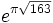On this page are notes from various courses I have taught over the last 20 years, primarily at Oxford, though in several cases these are based on courses I gave at Cambridge.

• Restriction and Kakeya phenomena (Cambridge, 2002: quite out of date in many places)
• Chapter 1 (Roth's Theorem)
• Chapter 2 (Sumsets)
• Chapter 3 (Freiman-Ruzsa theorem)
• Chapter 4 (Balog-Szemeredi-Gowers)
• Chapter 5 (Longer progressions, Gowers norms)
• Chapter 6 (Sum-product over Fp, exponential sums over subgroups) became these notes.
• Chapter 7 (Sum-product in C)
• Exercise sheets for the course: Sheet 1, Sheet 2, Sheet 3. Thanks to Sarah Penington for providing me with scanned and annotated copies of the old sheets, which I had lost.
• Ergodic theory (16 lectures). These are notes from the course C4.5 Ergodic Theory, which I have taught twice at Oxford. They cover the basic concepts and theorems of ergodic theory, and then turn to applications to combinatorial number theory, in particular Furstenberg's proof of Szemeredi's theorem for progressions of length 3. There are appendices on measure theory and basic Fourier analysis
• The Prime Number Theorem (16 lectures) These are the notes for the course C3.8 Analytic Number Theory, which I have taught twice at Oxford. They give a full proof of the prime number theorem via the explicit formula.
• Riemann Integration (8 lectures). These are notes for the course Analysis III: Integration at Oxford, which I have lectured three times. This is a rigorous introduction to the Riemann integral.
• Introduction to number theory (8 lectures). These are notes for ASO Number Theory at Oxford, which I have lectured three times. This is a first course in number theory, with topics including modular arithmetic, primitive roots, quadratic reciprocity and primality testing.
• Algebraic Number Theory (16 lectures). These are notes from when I lectured B3.4 Algebraic Number Theory at Oxford in 2020. They are a fairly gentle introduction to algebraic number theory with plenty of examples, with some additional material on binary quadratic forms and the class group. Exercise sheets for the course: Sheet 1, Sheet 2, Sheet 3, Sheet 4.
• Here are some odd bits and pieces that haven't found their way into published papers, blogs or the bin. Some of these were written over 15 years ago and haven't been updated since, so use at your own risk. Some are just quite standard bits of undergraduate material that I wrote up for one reason or another.

• Edinburgh Lecture Notes on Freiman's Theorem (2002)
• Progressions and Convex Geometry (2005, supplement to the above)
• Bourgain's bound for 3-term progressions (1999)
• Bourgain's work on arithmetic progressions in sumsets (2000)
• Heath-Brown-Szemeredi bound for 3-term progressions (1999)
•is exceptionally close to an integer (1998).
• An example proving that dense Freiman models of sets with small doubling need not exist in the nonabelian case (2007)
• The Hahn-Banach theorem (2011)
• A couple of standard facts about continued fractions (2012)
• Runge's theorem (2012)
• The Baire Category Theorem (2009)
• Review of Additive Combinatorics by Tao and Vu (2007)
• A very brief review of measure theory (2008)
• A quick primer on conditional expectation (2008)
• The Asymmetric Balog-Szemeredi-Gowers theorem (2016)